Function Repository Resource:

# ParetoListMinima

Find the Pareto-minimal points in a set of numeric vectors

Contributed by: Daniel Lichtblau
 ResourceFunction["ParetoListMinima"][points] gives the subset of points that are Pareto-minimal.

## Details and Options

Given a set of real-valued points in n-dimensional space, a point p={p1,,pn} is defined to be Pareto-minimal if no other point in the set q={q1,,qn} has q1pi for all 1in.
Pareto-minima are minimal elements with respect to the partial ordering induced by component-wise less-or-equal comparisons.
ResourceFunction["ParetoListMinima"] uses the Bentley-Clarkson-Levine algorithm.
The result can have points in a different order from their relative positioning in the input.

## Examples

### Basic Examples (1)

Find the Pareto minima of five points in 3D:

 In:=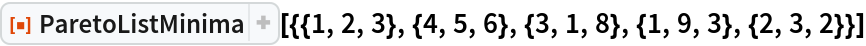Out=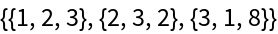### Scope (4)

Create a list of 20 random integer vectors in 3D:

 In:=Out=Compute the Pareto minima for this set:

 In:=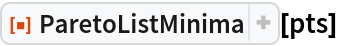Out=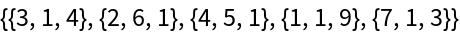Compute the Pareto minima for a set of 200 points in 2D:

 In:=Out=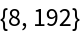Show the Pareto minima connected by segments, along with the other points:

 In:=Out=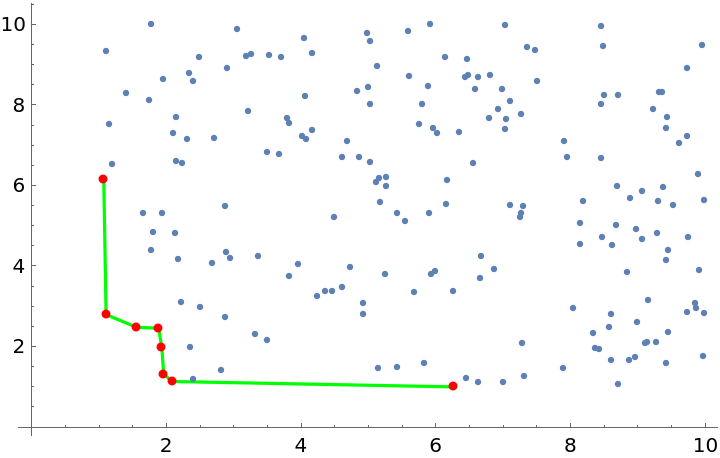Pareto-maximal points are defined in the same way as minimal points, except using GreaterEqual as the component-wise inequality. One can use ParetoListMinima in a simple way to compute the Pareto-maximal points:

 In:=Out=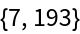Show the Pareto maxima connected by segments, along with the other points:

 In:=Out=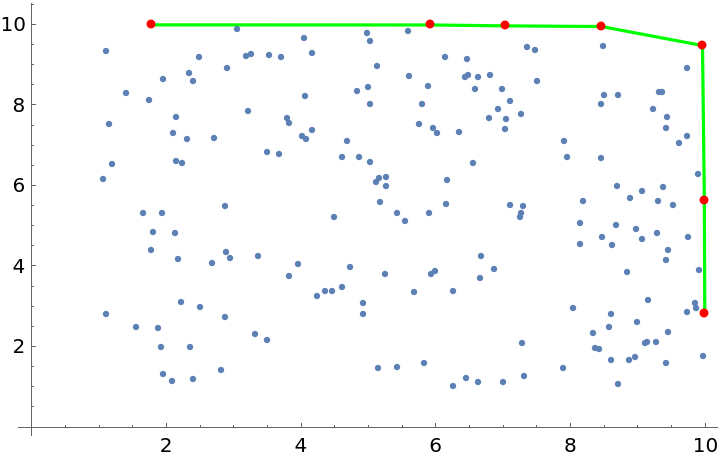Compute Pareto minima for 200 points in 3D:

 In:=Out=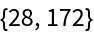Show the Pareto maxima, the planar mesh they define, and the non-minima above them:

 In:=Out=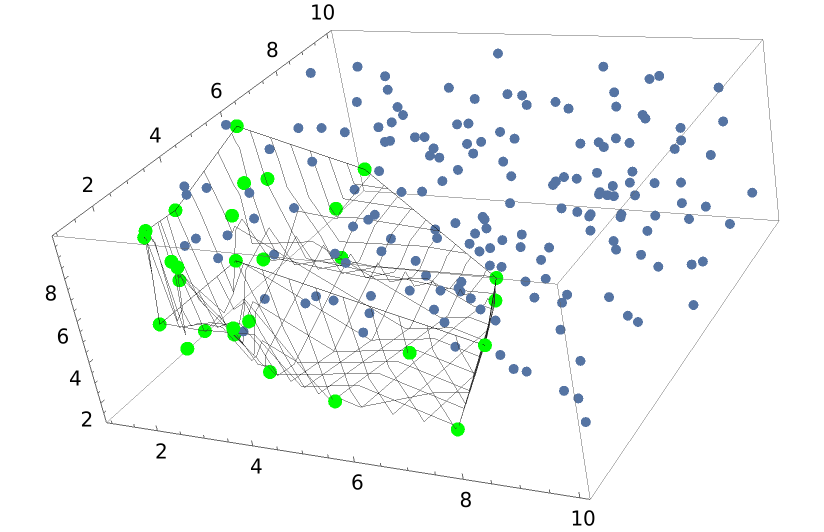## Version History

• 1.0.0 – 24 April 2020